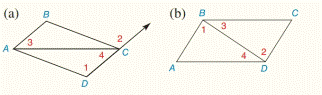Chapter 2.CR, Problem 1CR### Elementary Geometry for College St...

6th Edition
Daniel C. Alexander + 1 other
ISBN: 9781285195698

#### Solutions

Chapter
Section### Elementary Geometry for College St...

6th Edition
Daniel C. Alexander + 1 other
ISBN: 9781285195698
Textbook Problem
1 views

# If m ∠ 1 = m ∠ 2 , which lines are parallel?To determine

a)

To find:

The parallel lines.

Explanation

Given:

The given statement is,

m1=m2

Figure (1)

Approach:

The given statement is,

m1=m2

So,

12

1 and 2 are two congruent angles

To determine

(b)

To find:

The parallel lines.

### Still sussing out bartleby?

Check out a sample textbook solution.

See a sample solution

#### The Solution to Your Study Problems

Bartleby provides explanations to thousands of textbook problems written by our experts, many with advanced degrees!

Get Started

#### Find more solutions based on key concepts